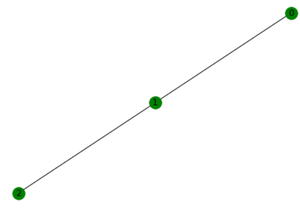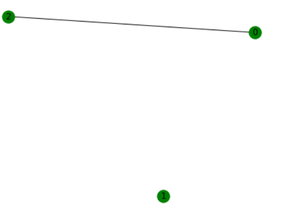Related Articles

# Visualizing the complement of a graph using networkx

• Last Updated : 13 Jan, 2021

Given a graph G complement of the graph is obtained by removing the edges contained in G from the complete graph having the same number of nodes as G.

Example:

Initial Graph G:G

The complement of G:G Complement

### Realizing Complement using Python :

We will use networkx.complement() function for our task

Syntax:

networkx.complement()

• Returns the complement of the graph object passed.
• The value returned is of the same type of the value passed i.e networkx graph object.
• G is the initial object passed.
• Although the complement is being created but no self-loops and parallel edges are created.

### Working of complement(G) function:

An edge (n,n2) where n is iterator used to iterate over G is added to complement graph if following conditions are met:

• n and n2 are not neighbours i.e n2 are in the adjacency list of n and vice versa.
• And n != n2.
• n and n2 both are part of G.

Approach:

• We will import networkx with an alias nx.
• Create a sample graph object G using path_graph() function.
• We will then call complement function passing G as an argument.
• We will capture the object returned by complement function in another object G_C.
• We will then call draw() function passing G_C as an argument which will display of the complement graph.

## Python3

 `# importing networkx module``import` `networkx as nx`` ` `# creating sample graph object``G ``=` `nx.path_graph(``3``)`` ` `# complement of G and saving in G_C``G_C ``=` `nx.complement(G)`` ` ` ` `# calling draw() to visualize the original graph``nx.draw(G, node_color``=``'Green'``, with_labels``=``True``)`` ` ` ` `# calling draw() to visualize the complement graph``nx.draw(G_C, node_color``=``'Green'``, with_labels``=``True``)`

Output:Original GraphComplement Graph

By using the complement() method all the edges in G were removed and all other possibilities were added to the complement of G and printed.

Attention reader! Don’t stop learning now. Get hold of all the important DSA concepts with the DSA Self Paced Course at a student-friendly price and become industry ready.  To complete your preparation from learning a language to DS Algo and many more,  please refer Complete Interview Preparation Course.

In case you wish to attend live classes with experts, please refer DSA Live Classes for Working Professionals and Competitive Programming Live for Students.

My Personal Notes arrow_drop_up